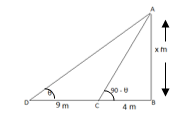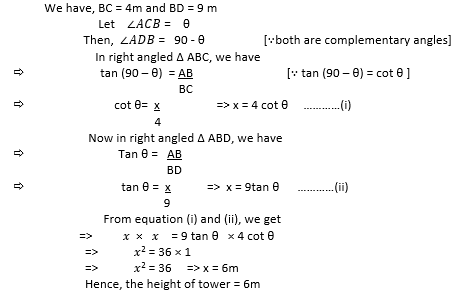# Chapter 9 Some Applications of Trigonometry

At Saralstudy, we are providing you with the solution of Class 10 Mathematics Some Applications of Trigonometry according to the latest NCERT (CBSE) Book guidelines prepared by expert teachers. Here we are trying to give you a detailed answer to the questions of the entire topic of this chapter so that you can get more marks in your examinations by preparing the answers based on this lesson. We are trying our best to give you detailed answers to all the questions of all the topics of Class 10th mathematics Some Applications of Trigonometry so that you can prepare for the exam according to your own pace and your speed.

Download pdf of NCERT Solutions for Class Mathematics Chapter 9 Some Applications of Trigonometry

Download pdf of NCERT Examplar with Solutions for Class Mathematics Chapter 9 Some Applications of Trigonometry

### Exercise 1 ( Page No. : 205 )

•  Q1 A circus artist is climbing a 20 m long rope, which is tightly stretched and tied from the top of a vertical pole to the ground. Find the height of the pole, if the angle made by the rope with the ground level is 30° (see Fig. 9.11). Ans: Let AB = hm be the height of pole and length of rope is 20 m tied from the          Top of tower AB and = 30o                                                                            In right   Δ ABC, we have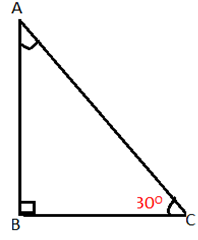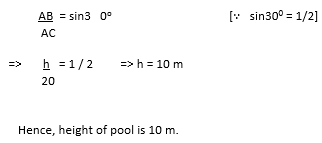Q2 A tree breaks due to storm and the broken part bends so that the top of the tree touches the ground making an angle 30° with it. The distance between the foot of the tree to the point where the top touches the ground is 8 m. Find the height of the tree. Ans: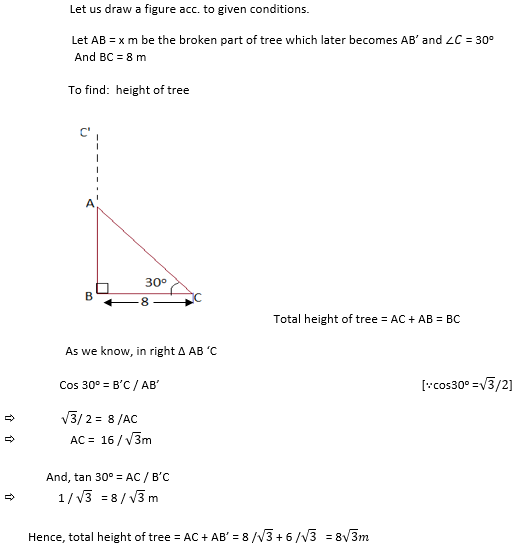Q3 A contractor plans to install two slides for the children to play in a park. For the children below the age of 5 years, she prefers to have a slide whose top is at a height of 1.5 m, andis inclined at an angle of 30° to the ground, whereas for elder children, she wants to have a steep slide at a height of 3m, and inclined at an angle of 60° to the ground. What should be the length of the slide in each case? Ans: (i) For Children below age of 5 years Height of slide = 1.5 m Angle of inclination of slide = 30o (ii) For Children below age of 5 years Height of slide = 3m Angle of inclination of slide = 60o Draw figure for both cases: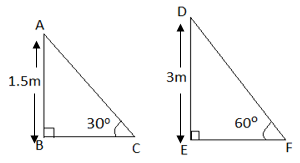In right Δ ABC, we have Sin 30o  =  AB / AC           =>     1/ 2  = 1.5 /AC                                                      [ sin30o =1/2] AC  =  3m In right Δ DEF, we have Sin 60o  = DE /DF                 =>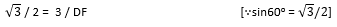DF  =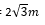Q4 The angle of elevation of the top of a tower from a point on the ground, which is 30 m away from the foot of the tower, is 30°. Find the height of the tower. Ans: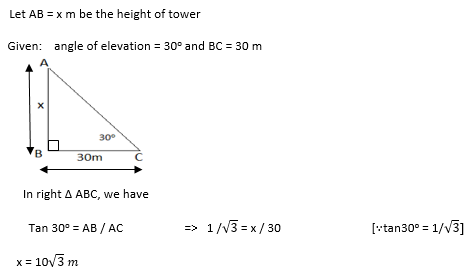Q5 A kite is flying at a height of 60 m above the ground. The string attached to the kite is temporarily tied to a point on the ground. The inclination of the string with the ground is 60°. Find the length of the string, assuming that there is no slack in the string. Ans: Draw a figure according  to given conditions,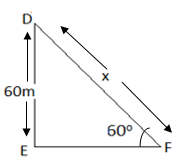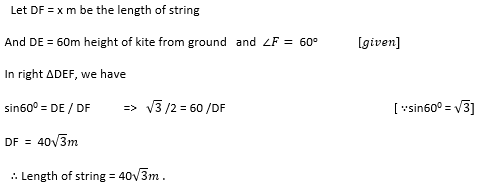Q6 A 1.5 m tall boy is standing at some distance from a 30 m tall building. The angle of elevation from his eyes to the top of the building increases from 30° to 60° as he walks towards the building. Find the distance he walked towards the building. Ans: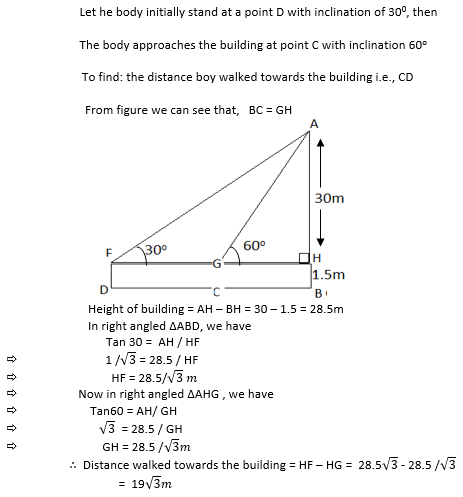Q7 From a point on the ground, the angles of elevation of the bottom and the top of a transmission tower fixed at the top of a 20 m high building are 45° and 60° respectively. Find the height of the tower. Ans: Let AB = x m be the height of transmission tower          BC = 20 m height of building       (given)          Angle of elevation from top of tower = 600           Angle of elevation from bottom of tower = 45o           To find : height of tower i.e., AB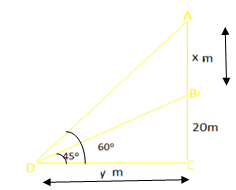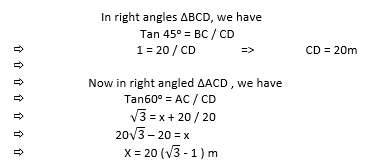Q8 A statue, 1.6 m tall, stands on the top of a pedestal. From a point on the ground, the angle of elevation of the top of the statue is 60° and from the same point the angle of elevation of the top of the pedestal is 45°. Find the height of the pedestal. Ans: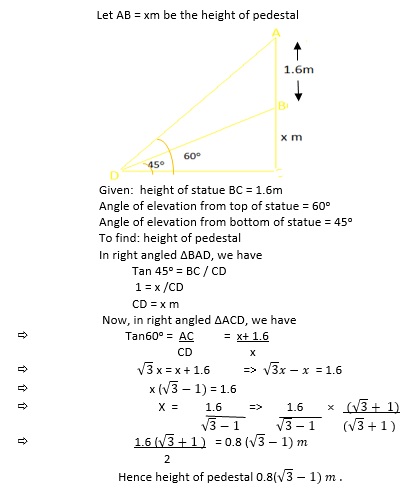Q9 The angle of elevation of the top of a building from the foot of the tower is 30° and the angle of elevation of the top of the tower from the foot of the building is 60°. If the tower is 50 m high, find the height of the building. Ans: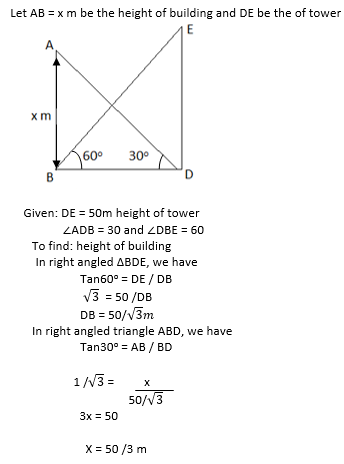Q10 Two poles of equal heights are standing opposite each other on either side of the road, which is 80 m wide. From a point between them on the road, the angles of elevation of the top of the poles are 60° and 30°, respectively. Find the height of the poles and the distances of the point from the poles. Ans: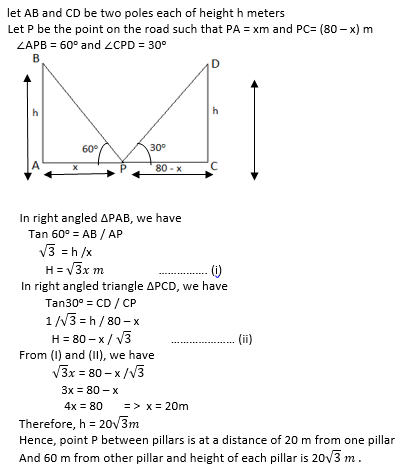Q11 A TV tower stands vertically on a bank of a canal. From a point on the other bank directly opposite the tower, the angle of elevation of the top of the tower is 60°. From another point 20 m away from this point on the line joing this point to the foot of the tower, the angle of elevation of the top of the tower is 30° (see Fig. 9.12). Find the height of the tower and the width of the canal. Ans: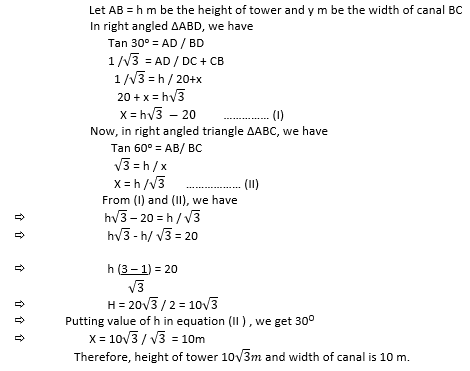Q12 From the top of a 7 m high building, the angle of elevation of the top of a cable tower is 60° and the angle of depression of its foot is 45°. Determine the height of the tower. Ans: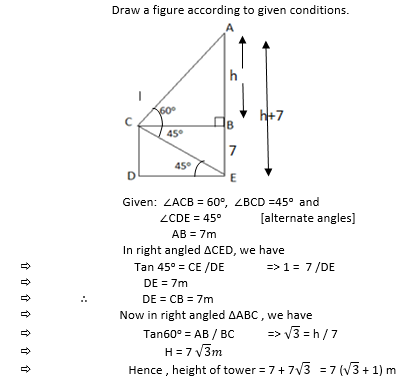Q13 As observed from the top of a 75 m high lighthouse from the sea-level, the angles of depression of two ships are 30° and 45°. If one ship is exactly behind the other on the same side of the lighthouse, find the distance between the two ships. Ans: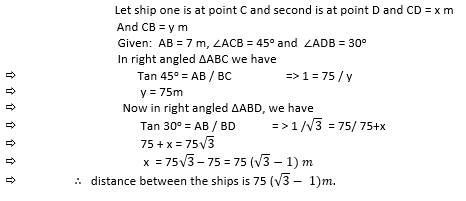Q14 A 1.2 m tall girl spots a balloon moving with the wind in a horizontal line at a height of 88.2 m from the ground. The angle of elevation of the balloon from the eyes of the girl at any instant is 60°. After some time, the angle of elevation reduces to 30° (see Fig. 9.13). Find the distance travelled by the balloon during the interval. Ans: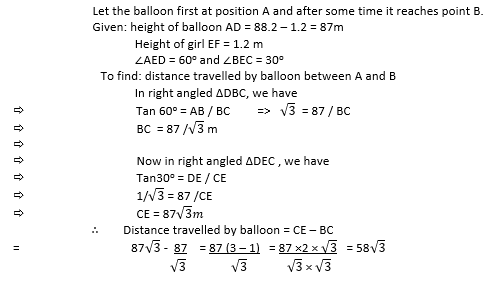Q15 A straight highway leads to the foot of a tower. A man standing at the top of the tower observes a car at an angle of depression of 30°, which is approaching the foot of the tower with a uniform speed. Six seconds later, the angle of depression of the car is found to be 60°. Find the time taken by the car to reach the foot of the tower from this point. Ans: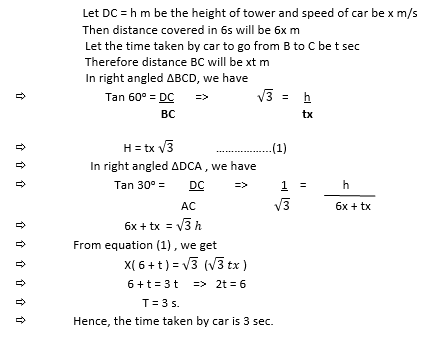Q16 The angles of elevation of the top of a tower from two points at a distance of 4 m and 9 m from the base of the tower and in the same straight line with it are complementary. Prove that the height of the tower is 6 m. Ans: Let the height of tower AB = x m